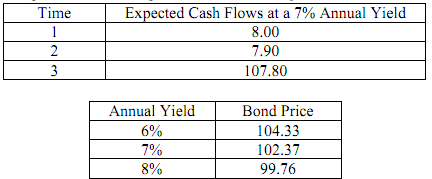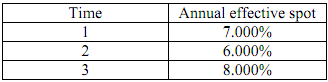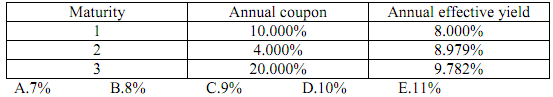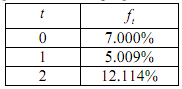### Theory of interest - term structure of interest rates

Assignment Help Accounting Basics
##### Reference no: EM13494

1.  The current price of a bond is \$114.72 and the current yield is 6.00%. The modified duration of the bond is 7.02. Use the modified duration to estimate the price of the bond if the yield increases to 6.10%.

A.114    B.120    C.210    D.220    E.300

2.  A two-year bond has 8% annual coupons payable semiannually. The bond's yield is 10% compounded semiannually. Calculate the modified duration of the bond.

A.0.8    B.1.0    C.1.5    D.1.8    E.2.0 ok

3.  A 22-year bond pays 7% annual coupons and has a current price of \$81.12. The annual effective yield on the bond is 9%. The Macaulay duration of the bond is 10.774. Estimate the new price if the yield falls to 8.95%.

A.76    B.78    C.80    D.82    E.84

4.  A 15-year mortgage is repaid with level monthly payments. The yield is 12% compounded monthly. Calculate the Macaulay duration of the mortgage.

A.3.2    B.5.4    C.7.1    D.9.7    E.10.3

5.  A 20-year bond yielding 9% has a price of \$127.79. If the bond's yield falls to 8.75%, then the price of the bond will increase to \$130.65. If bond's yield increases to 9.25%, then the price of the bond will fall to \$125.02. Calculate the effective duration of the bond.

A.8.6    B.8.7    C.8.8    D.8.9    E.9.0

6.  A five-year bond with a coupon of 6.7% pays coupons semiannually. It is currently yielding 6.4%. Its current price is \$101.2666. If the bond's yield increases by 10 basis points, then its price falls to \$100.8422. If the bond's yield falls by 10 basis points, then its price rises to \$101.6931. Calculate the effective convexity of the bond.

A.15.67  B.17.75  C.18.52  D.19.32  E.20.74

7.  The modified duration of an 8-year bond is 5.35 and its convexity is 39.19. Estimate the percentage change in the price of the bond if its yield increases by 63 basis points.

A. -3.29%  B.-1.09%  C.1.09%  D.3.29%  E.5.14%

8.  An insurance company has committed to make a payment of \$100,000 in 5 years. The insurance company can fund this liability only through the purchase of 4-year zero-coupon bonds and 10-year zero-coupon bonds. The annual effective yield for all assets and liabilities is 12%. Determine how much the bank should invest in the 4-year zero-coupon bond in order to immunize its position.

A.26401  B.26933  C.47286  D.47813  E.48005

9.  You are given the following information with respect to a callable bond:The current yield is 7%.

Calculate the ratio of the modified duration to the effective duration of this bond.

A.1.01   B.1.17    C.1.32    D.1.38   E.1.50

10. The current price of a bond is 100. The derivative of the price with respect to the yield to maturity is -700. The yield to maturity is 8%.

Calculate the Macaulay duration.
A.8    B.9    C.10    D.11    E.12

11. Given the following annual effective spot rates, find the present value of a 3-year bond paying 15% annual coupons and having a par value of \$100.12. Given the following yields and coupons for bonds with \$100 of par value, determine the 2-year annual effective spot rate.13. Given the following table of forward rates, find the present value of a 3-year bond paying 15% annual coupons and having a par value of \$100.

A.118.66  B.120.24  C.132.86  D.144.12  E.151.97### Write a Review